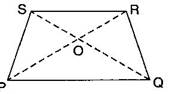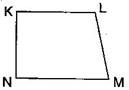### Basic Geometrical Ideas-Solutions Ex-4.5

CBSE Class –VI Mathematics
NCERT Solutions
Chapter 4
Basic Geometrical Ideas (Ex. 4.5)

Question 1. Draw a rough sketch of a quadrilateral PQRS. Draw its diagonals. Name them. Is the meeting point of the diagonals in the interior or exterior of the quadrilateral?Diagonal PR and diagonal SQ meet at O, which is inside the quadrilateral.

Question 2. Draw a rough sketch of a quadrilateral KLMN. State:
(a) Two pairs of opposite sides.
(b) Two pairs of opposite angles.
(c) Two pairs of adjacent sides.
(d) Two pairs of adjacent angles.
Answer: (a) Pair of opposite sides: KL and MN, KN and LM
(b) Pair of opposite angles: $\mathrm{\angle }$K and $\mathrm{\angle }$M, $\mathrm{\angle }$L and $\mathrm{\angle }$N
(c) Pair of adjacent sides: KN and NM, KL and LM
(d) Pair of adjacent angles: $\mathrm{\angle }$K and $\mathrm{\angle }$N, $\mathrm{\angle }$L and $\mathrm{\angle }$MQuestion 3. Investigate:
Use strip and fasteners to make a triangle and a quadrilateral.
Try to push inward at any one vertex of the triangle. Do the same to the quadrilateral.
Is the triangle distorted? Is the quadrilateral distorted? Is the triangle rigid?
Why is it that structures like electric towers make use of triangular shapes and not quadrilateral?
Answer: No, the triangle is not distorted but the quadrilateral is distorted and also the triangle is rigid.
Structures like electric towers make use of triangular shape so that they could not be distorted and they could be rigid.